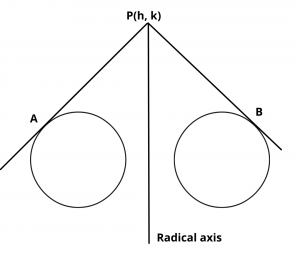# Radical Axis of Two circles – Definition and Formula

Here you will learn what is the formula to find the equation of radical axis of two circles.

Let’s begin –

## Radical Axis of Two Circles

Definition : The locus of a point, which moves in such a way that the length of tangents drawn from it to the circle are equal and is called the radical axis.

If two circles are –$$S_1$$ = $$x^2 + y^2 + 2g_1x + 2f_1y + c_1$$ = 0

$$S_2$$ = $$x^2 + y^2 + 2g_2x + 2f_2y + c_2$$ = 0

Let P(h, k) is a point and PA, PB are length of two tangents on the circles from point P,

Then from definition –

$$\sqrt{h^2 + k^2 + 2g_1h + 2f_1k + c_1}$$ = $$\sqrt{h^2 + k^2 + 2g_2h + 2f_2k + c_2}$$

$$\implies$$  $$2(g_1 – g_2)h + 2(f_1 – f_2)k + c_1 – c_2$$ = 0

$$\therefore$$   Locus of (h, k)

$$2x(g_1 – g_2)$$ + $$2y(f_1 – f_2)$$ + $$c1 – c_2$$ = 0

$$S_1 – S_2$$ = 0

which is the equation of radical axis.

Also Read : Pole and Polar of a Circle Equation

To get the radical axis of three circle $$S_1$$ = 0, $$S_2$$ = 0, $$S_3$$ = 0 we have to solve any two
$$S_1 – S_2$$ = 0, $$S_2 – S_3$$ = 0, $$S_3 – S_1$$ = 0